Pure and Applied Mathematics Journal
Volume 5, Issue 6, December 2016, Pages: 192-204

The Singular Function Boundary Integral Method for the 2-D and 3-D Laplace Equation Problems in Mechanics, with a Boundary Singularity

Department of Mathematics and Statistics, University of Cyprus, Nicosia, CyprusMiltiades C. Elliotis. The Singular Function Boundary Integral Method for the 2-D and 3-D Laplace Equation Problems in Mechanics, with a Boundary Singularity. Pure and Applied Mathematics Journal. Vol. 5, No. 6, 2016, pp. 192-204. doi: 10.11648/j.pamj.20160506.14

Received: October 3, 2016; Accepted: October 15, 2016; Published: November 10, 2016

Abstract: In this study the Singular Function Boundary Integral Method (SFBIM) is implemented in the case of a planar elliptic boundary value problem in Mechanics, with a point boundary singularity. The method is also extended in the case of a typical problem of Solid Mechanics, concerning the Laplace equation problem in three dimensions, defined in a domain with a straight edge singularity on the surface boundary. In both the 2-D and 3-D cases, the general solution of the Laplace equation is approximated by the leading terms (which contain the singular functions) of the local asymptotic solution expansion. The singular functions are used to weight the governing equation in the Galerkin sense. For the 2-D Laplacian model problem of this study, which is defined over a domain with a re-entrant corner, the resulting discretized equations are reduced to boundary integrals by means of Green’s second identity. For the 3-D model problem of this work, the volume integrals of the discretized equations are reduced to surface integrals by implementing Gauss’ divergence theorem. The Dirichlet boundary conditions are then weakly enforced by means of Lagrange multipliers. The values of the latter are calculated together with the singular coefficients, in the 2-D case or the Edge Flux Intensity Functions (EFIFs), in the 3-D model problem, which appear in the local solution expansion. For the planar problem, the numerical results are favorably compared with the analytic solution. Especially for the extension of the method in three dimensions, the preliminary numerical results compare favorably with available post-processed finite element results.

Keywords: Laplace Equation, Boundary Singularity, Straight Edge Singularity, Singular Coefficients, Edge Flux Intensity Functions, Singular Function Boundary Integral Method

1. Introduction

In the past few decades, many numerical methods have been proposed for the solution of 2-D elliptic boundary value problems in Mechanics, with a boundary singularity. The lack of adequate accuracy and poor convergence, were some of the difficulties which appeared in the attempt of many researchers to solve this kind of problems. Remedies used were special mesh-refinement schemes, multigrid methods, singular elements, p/hp finite elements and many other techniques (e.g. [1-3, 5, 24, 25]). An extensive survey of the treatment of singularities in elliptic 2-D boundary value problems is provided in . Of course, in the absence of a boundary singularity, there are many other efficient numerical techniques available in the literature (e.g. [1, 2, 4, 22, 29]) to be used for the solution of problems in Mechanics.

For the 2-D problems with a boundary singularity there are more sophisticated techniques in the literature, than those mentioned above, which incorporate the form of the local asymptotic expansion. For example, in  a singular finite element method is developed for Stoke’ s flow problems, in which special elements are employed, in the neighborhood of the singularity, with which the radial form of the local expansion is utilized, in order to resolve the convergence difficulties and improve the accuracy of the global solution. The interest in such two-dimensional Laplace equation problems with a boundary singularity , is motivated by the need to compute the singular coefficients of the local solution expansion which, in the area of Solid Mechanics, normally represents the Airy stress function Φ(r,θ) and expressed as follows: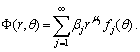(1)

In the above asymptotic expansion, μj are the eigenvalues and fj(θ) are the eigenfuntions of the problem and they are known. The polar coordinates (r, θ) have as a center the singular point. Singular coefficients βj are the primary unknowns in this kind of problems. They are also known as generalized stress intensity factors (GSIFs). These are determined by the boundary conditions on the boundary parts which are away from the singularity. They are of significant importance in many applications in the area of Fracture Mechanics . Thus, after the calculation of the leading singular coefficients βj, the approximation of Φ(x,y) is known (by converting into Cartesian coordinates) and the approximate stress state, at any position, is found from the known expressions: σxx=2Φ/y2, σyy=2Φ/x2 and σxy=-2Φ/xy.

In the past two decades, the Singular Function Boundary Integral Method (SFBIM) was developed [9-14, 17, 19], which belongs to the general group of collocation methods  and with which the unknown singular coefficients are calculated directly. Thus, the method gives directly the approximation of the Airy stress function (for solids) or the stream function (for fluids) in planar Laplacian and biharmonic problems. Basically, for the implementation of the SFBIM to solve 2-D problems with a boundary singularity, it is required that the solution is approximated by the leading terms of expansion (1). The Dirichlet conditions, on the boundary parts, which are away from the singularity, are enforced by means of Lagrange multipliers. The method has been tested on standard elliptic problems exhibiting exponential convergence and high accuracy with respect to the number of singular functions. This behavior of the method has been theoretically proved in . The results obtained in the 2-D case have encouraged the extension of the method in 3-D problems with a straight-edge singularity [6, 13].

The 2-D model problem, treated in this study, is a Laplacian problem defined over a disk, with a boundary Ω on which a quarter of a circle is missing and thus a boundary singularity at the centre, is created. This could be a heat transfer problem in two dimensions, but in this study it is a plane stress problem of a perfectly elastic solid, on which the interest is to find the unknown singular coefficients βj, of expansion (1). As for the 3-D case, we consider a Laplacian boundary value problem in a 3-D domain. This is a model for an elastic cylindrical body, having a typical V-notch, made of an isotropic material which obeys to Hooke’s Law and which is subjected to certain physical conditions. The form of the local solution depends on the geometry in the neighbourhood of the straight-edge singularity and on the boundary conditions of the boundary parts which share the singularity. Also, the local solution is characterized by the presence of certain eigenpairs (arising from the 2-D problem) and the so-called Edge Flux Intensity Functions (EFIFs), which are the primary unknowns in the 3-D case . The interest in such a problem is motivated by the need to compute generalized stress intensity functions for the V-notched solids loaded by static loads. For the solution of this class of 3-D problems, few methods have been proposed so far, such as the J-integral method , the B- and H-integral methods , and more recently the methods by Costabel et al.  and Yosibash et al. [32-35] and Zaltzman , in which the EFIFs are computed by means of a post-processing procedure in a p-version finite element scheme.

Based on  and , the solution u of the 3-D Laplace equation, in a domain with an edge singularity, may be written in terms of cylindrical coordinates (r, θ, z) as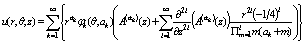(2)

In the above expansion, ακ R and φκ(θ,ακ) is the known eigenpair, of the two-dimensional Laplace operator. The functions φκ(θ,ακ) are analytic in θ. The functions Α(ακ)(z) are the EFIFs. These are functions of z and for a large class of problems they are the primary unknowns. In Solid Mechanics , function u represents the first stress invariant (i.e. u=σii).

In the SFBIM, the solution is approximated by the leading terms of the asymptotic expansion (1) for the 2-D case, or the asymptotic expansion (2) for the 3-D case. The leading terms of these expansions are also used to discretize the governing differential equation in the Galerkin sense. The discretized equations are reduced to boundary integrals by means of the Green’s second identity, for the 2-D case, or the Gauss divergence theorem, for the 3-D case. A particular feature of the SFBIM is that the Dirichlet conditions are weakly enforced by means of Lagrange multipliers. In two-dimensional problems the coefficients of the asymptotic expansion are constants; these are calculated directly by the SFBIM. As we have already mentioned above, in three dimensions the EFIFs are functions of the axial direction. In the present approach, these are approximated by polynomials the coefficients of which are primary unknowns and are calculated directly by the method.

The outline of the rest of this paper is as follows: in Section 2 we present both a 2-D Laplace equation problem, with a point boundary singularity and a 3-D Laplace equation problem with a straight-edge singularity. The asymptotic local solution expansion is also given for each one of these problems. In Section 3 the formulation of the SFBIM is presented both in two and three dimensions. Numerical results are given in Section 4 together with comparisons between the results obtained with the SFBIM and the exact solution. Especially for the extension of the method in three dimensions, the numerical results obtained are compared with post-processed finite element results available in the literature . Finally, conclusions derived from this study are summarized in Section 5.

2. Governing Equation and Local Solution in 2-D and 3-D

2.1. The Planar Model Problem of the Laplace Equation

The 2-D model problem is a problem of a Laplace equation defined over a domain Ω. This domain is a very thin disk with radius R and with a re-entrant corner on its boundary, which has its tip on the center O of the disk (Figure 1). Thus, at point O there is a boundary singularity. Boundary parts OA and OB, which share the singularity, intersect vertically at O and each one has the Neumann condition: Φ/n=0. Circular part AB has the Dirichlet condition: Φ=f(θ). The material of the disk is isotropic and linearly elastic. Thus, basically, with the specific geometry and boundary conditions of this problem we need to find an expression for the Airy stress function Φ(r,θ), which is the solution of the Laplace equation. The values of Young’s modulus and of Poisson’s ratio are not necessary for the solution of the problem. It must be mentioned that this model problem belongs to the general class of problems of two-dimensional plates with geometric singularities on the boundary.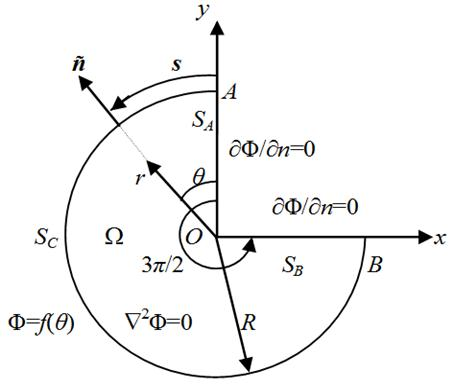Fig. 1. Geometry and boundary conditions of the 2-D Laplacian model problem.

The planar model problem of the Laplace equation, illustrated by Figure 1 is the following: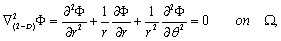(3)

with the boundary conditions(4)

For this specific model problem the Dirichlet condition on the boundary SC has the following trigonometric expression:

Φ(θ)=(2/5)R2/3cos(2θ/3)+(4/7)R4/3cos(4θ/3)

where R is the radius of boundary SC.

Using the separation of variables approach and the boundary conditions on SA and SB it can be easily found that the solution around the singular point is found to be Φ(r,θ)=βrμjcos(μjθ). Furthermore, by employing the principle of superposition we obtain the local solution expansion, which is expressed in terms of polar coordinates (r,θ), centered at O: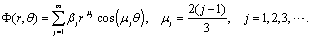(5)

Also, by considering the 2π-periodicity property of the trigonometric functions, singular coefficients βj can be calculated as Fourier coefficients by using Euler’s formula. So, the exact solution of the 2-D model problem, defined by (3) and (4), is found to be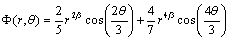on Ω.(6)

Using the maximum principle theorem in its weak form (e.g. ) it can be shown that the above solution of the 2-D problem is unique.

2.2. The 3-D Model Problem of the Laplace Equation

The geometry and the boundary conditions of the 3-D model problem of the Laplace equation, are illustrated in Figure 2. The domain Ω is bounded by a cylindrical surface SC around the z-axis, a circular sector SD lying on the xy-plane, a flat circular sector SE perpendicular to the z-axis and at a distance L from SD (here L=2) and two flat boundaries SA and SB (one parallel to the xz plane and the other parallel to the yz plane) intersecting vertically on the z-axis and thus creating a V-notch, which is the straight-edge singularity of this problem.

This solid body is made of an isotropic linearly elastic material and has no voids inside. For the specific geometry and boundary conditions of this model problem, the solution u, which, as we have already mentioned, normally represents the first stress invariant, is independent of any combination of values of the Young modulus E and the Poisson ratio ν. As we know, these are important parameters for the calculation of stresses and strains in linearly elastic materials which obey to Hooke’s law.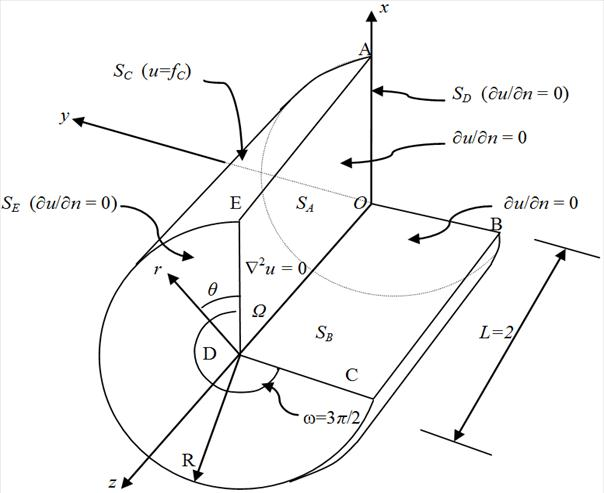Fig. 2. Geometry and boundary conditions of the 3-D Laplacian model problem.

This problem is a version of a more general problem which was first suggested by Yosibash et al.  and is as follows: Find u such that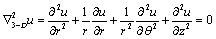in Ω,(7)

with the following boundary conditions(8)

where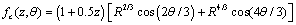Naturally, the Laplace equation (7) is expressed in cylindrical co-ordinates, which are more convenient for both the analytical and numerical treatment of the problem.

In  it was shown that the solution u, of the three-dimensional general Laplace equation problem with a governing equation 23-D u = 0 in Ω, such that the boundary conditions (8) hold, is obtained by augmenting the 2-D solution of the Laplace equation. Thus, according to , the solution in the neighborhood of the edge singularity, is given by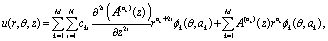(9)

where (r,θ,z)Ω are cylindrical coordinates, with the z-axis along the boundary straight-edge singularity. Functions A(ak)(z) are the EFIFs, which were described earlier. In this work they are chosen to be polynomials of degree Np and with unknown coefficients ak,j as follows: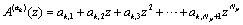(10)

The constants cki are given by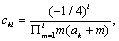and the eigenfunctions φk and eigenvalues αk are given by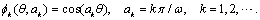(11)

Here, ω is the external angle defined by the flat boundaries ODEA and ODCB as shown in Figure 2; in the present work ω = 3π/2. Moreover, for the model problem of Figure 2, the first two EFIFs are known and they are of the form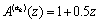for k=1 or 2

We must emphasize, at this stage, that the model problem of the 3-D case is selected in a way that the exact solution is known and given by a (finite) sum. Investigation of the uniqueness of this solution is similar with that of the 2-D case. Furthermore, since the only two non-zero EFIFs are polynomials, the accuracy of the EFIFs, computed by our method, can be measured by simply comparing the polynomial coefficients of the true and approximate EFIFs. In general, however, the true EFIFs are not always polynomials and the accuracy of the EFIFs computed by the numerical method, would need to be measured using some appropriate function norm.

3. The Singular Function Boundary Integral Method

3.1. The SFBIM in the 2-D Case

The first step of the method, in both the planar and the three-dimensional problems, is the approximation of the local solution expansion with its leading terms. Thus, for the 2-D problem of Figure 1, expansion (5) is approximated by its first Na terms: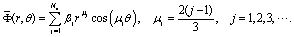(12)

The above series can also be written, more simply, as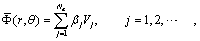(13)

where Vj =rμjcos(μjθ). Functions Vj are called singular functions and from now on, they are going to play an important role in the development of the formulation of the method. Note that these functions satisfy the governing equation and the boundary conditions along SA and SB.

Next step is to weight the governing equation by the singular functions Vi, in the Galerkin sense. Thus, we obtain the first set of discretized linear equations: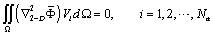(14)

So, we have our first set of Na discretized equations. Next, we apply Green’s second identity by considering that both the approximation of Φ and the singular functions Vi satisfy the Laplace equation and we obtain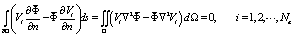(15)

The integrand of the first integral in (15) is equal to zero along boundary parts SA and SB, because there it is Φ/n=0 and Vi/n=0. Thus, the system of discretized equations (15) is simplified further to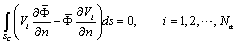(16)

For boundary part SC the Dirichlet boundary condition is imposed by employing the Langrange multipliers function which is expanded in terms of quadratic basis functions Mj as follows: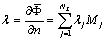(17)

In the above expansion, λj are the so-called Lagrange multipliers which are auxiliary parameters and they are additional unknowns in our problem. As we will see, they are calculated together with singular coefficients βj after solving the complete system of equations. The quadratic basis functions Mj, in expansion (17), are defined as follows:

For j odd(18)

and for j even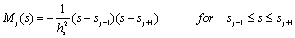(19)

In expressions (18) and (19) hs is the mesh-width, which is constant in this approach.

The normal derivative Vi/n, which appears in (16), is expressed as follows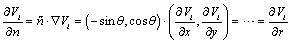(20)

where is the normal unit vector on boundary SC (see Figure 1). Furthermore, using (20) the normal derivative of Vi is given by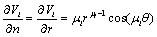(21)

which is an easy expression to be used in calculations.

Before we proceed further, it is more convenient to change, in all the above formulation, variable s with variable θ by considering that θ=s/R (Figure 1). Now, there is one more step before we have our complete system of linear equations. So, according to the SFBIM the Dirichlet condition on SC must be weighted with the basis functions Mi. Thus, finally, the discretized linear equations (16) take the form of the following system of Na+Nλ discretized equations:(22)

The integrands in (22) are not singular and integrations take place away from the singularity. If we substitute the approximation of Φ and function λ in (22), with their expressions in (13) and (17), respectively, then the system of equations (22) can further be written as(23)

The above system of linear equations can also be expressed in matrix form as follows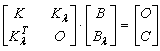(24)

or

K.b=c                                        (25)

where K is called stiffness matrix. It is a symmetric matrix but it becomes singular when Na < Nλ.

3.2. The SFBIM in the 3-D Case

In the SFBIM the solution of the problem is approximated by the leading terms of the local solution expansion given by (9). Thus, in (9) we employ the first Na terms and we substitute the EFIFs with their polynomial expression given by (10). Then the approximate solution is written as follows: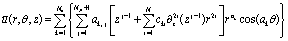(26)

where N (which also appears in (9)) is an additional parameter that allows us to ensure that the approximation of u satisfies the 3-D Laplace equation, by selecting it according to the restriction Np<2N+1 (see Appendix). We note that, in principle, N could be taken to be infinity since after all, the sum would terminate after a finite number of terms due to the fact that we are differentiating a polynomial of degree Np.

Following the notation used in previous applications of the SFBIM (e.g. [9-14]), the above expansion is written as follows: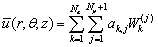(27)

In the above expression the coefficients ak,j will be referred to as singular coefficients and they are the coefficients of the polynomial expression (10) of the EFIFs. The functions Wk(j) are the singular functions (the same name was adopted for the 2-D approach) and in this case they have the form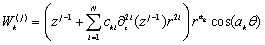(28)

It is easy to verify that 23-D (Wk(j))=0 in domain Ω and that the Wk(j)’s satisfy exactly the boundary conditions on SA and SB (see Appendix).

As in the 2-D case, we weight the governing equation by the singular functions Wk(j) in the Galerkin sense. This gives the first Na(Np+1) discretized equations: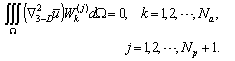(29)

Recalling that Wk(j) satisfies the governing equation and using Gauss’ divergence theorem we obtain(30)

where Ω=SASBSCSDSE. Since the singular functions Wk(j) satisfy exactly the boundary conditions on SA and SB and considering the boundary conditions (8), the boundary integral in (30) is identically zero along SA and SB. The discretized equations (30) then become(31)

As in the 2-D approach, the Dirichlet condition on the cylindrical boundary SC, which is away from the singularity, is imposed by means of a Lagrange multiplier function λ, which is expanded in terms of basis functions Mi(θ,z):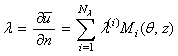on  SC,       (32)

where Nλ is the number of the discrete Lagrange multipliers λ(i) on SC. The nodal values of λ appear as additional unknowns in the problem. The additional Nλ required equations are then obtained by weighting the Dirichlet boundary condition on SC by the bilinear basis functions Mi(θ,z)in the Galerkin sense. Thus, in the end, we obtain the following linear system of (Np+1)Na+Nλ discretized equations:(33)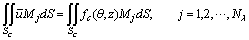(34)

Integration in the neighborhood of the point singularities O and D must be avoided. Thus, the EFIFs should be of degree equal to one or zero, for this problem (i.e. Np=1 or Np=0), in order to force the surface integrals on SC and SD to vanish. Therefore, by choosing a value of Np less or equal to 1 and by substituting the approximation of u and of the Lagrange multiplier function λ, with their expressions in (27) and (32), respectively, equations (33) and (34) take the form(35)(36)

It should be noted that, as in the 2-D case, the integrands in the above equations are non-singular and that all integrations are carried out far from the boundaries SA and SB and the points O and D causing the edge singularity. The surface integrals in (35) and (36) are two-dimensional integrals and are estimated using standard techniques, such as Gauss-Legendre quadrature. For example, if we use a 4-point rule the first of the integrals in equations (33) is estimated as follows:whereHere, Lz (l) and Lθ (l) are the dimensions of each element (l) along directions z and θ, respectively and NE is the number of boundary elements employed on the surface SC.

The system of discretized linear equations, (35) and (36), can be written in block form as follows:

K.aλ=C                               (37)

or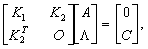(38)

where the vector A contains the unknown coefficients ak,j of the approximation of the EFIFs and the vector Λ contains the unknown (discrete) Lagrange multipliers λ(i). Clearly, the stiffness matrix K is symmetric. As in the case of 2-D problems, the number (Νp +1)Na of the unknown coefficients ak,j should be greater than or equal to the number of Lagrange multipliers Nλ, since the matrix Κ becomes singular when (Νp +1)Na< Nλ.

4. Numerical Experiments

4.1. Numerical Results for the 2-D Problem

Expansion (17) indicates clearly that the Lagrange multiplier function λ, which is used to impose the Dirichlet boundary condition on SC, has specific numerical values λ(j) at positions j which are chosen according to a selected subdivision of SC into boundary elements. For the 2-D model problem (Figure 1), boundary SC is subdivided into NE elements of equal size. Therefore, the number of Lagrange multipliers is Nλ=2NE+1.

The integrals in (23) are calculated numerically by subdividing each quadratic element into 10 subintervals and using a 15-point Gauss-Legendre quadrature over each subinterval. Since the stiffness matrix is symmetric only the elements which are on or above its principal diagonal are calculated. Now, in order to obtain the optimum combination of Na and Nλ, several series of runs have been made. Previous applications of the method in 2-D problems [9-14], indicated that Nλ should be large enough. This means that we must have an adequate number of elements on the boundary which will also help in having a better accuracy in numerical integration. However, Nλ should be smaller than or equal to Na in order to avoid ill-conditioning of the stiffness matrix. In general, very high values of Na are also avoided because although double precision is used in the codes, the computer accuracy cannot handle the contributions of the higher-order singular functions, which become very small for r < 1 or very large for r > 1. For the specific 2-D problem, the radius of the disk was chosen to be R=1. Hence, we do not have the phenomena described above. Thus, Nλ was varied from 3 to 15 and Na was varied from 13 to 30. After performing calculations within this range of combinations of values for Na and Nλ, it was observed that convergence occurs when Na=Nλ=13 (optimum choice).

Table 1 presents the convergence in the values of the four leading coefficients, with respect to the number of Lagrange  multipliers Nλ and for Na=13. As in previous implementations of the method [9-14], one may observe that the values of singular coefficients converge rapidly with Nλ. In fact, in  a theoretical analysis of the method proved algebraic convergence in Nλ. Also, very accurate estimates are obtained. Clearly, since the exact values of the leading singular coefficients of expansion (5) are β1=0, β2=2/5=0.40000000, β3=4/7≈0.571428571428571, β4=0 and βj=0, for j≥5, we can see, from Table 1, how fast convergence is achieved at Nλ=13.

The values of the leading singular coefficients calculated for Nλ=13 and various values of Na are shown in Table 2. Extremely fast convergence with respect to Na is observed (much faster than in previous implementations) and extremely accurate estimates are obtained. In fact, the leading singular coefficients converge from the very beginning. For Na>13 the solution starts to deteriorate. This is because the size of the system increases and thus the number of numerical calculations is increased together with the numerical errors which, of course, are not avoidable at the calculation of the matrix elements or at the numerical inversion of the stiffness matrix of the model problem. For the optimum combination Na=Nλ=13, the plot of λ as a function of variable θ, is shown in Figure 3. As expected, it has the form of a cosine trigonometric function because on SC we have Vi/r=μirμi-1cos(μiθ). In this graph the curve is smooth and free from oscillations.

In Table 3 the converged values of the singular coefficients, calculated for the optimal choices Na=Nλ=13 and R=1, are presented. The values of this table indicate clearly that the contribution of the higher order terms, vanishes immediately for this 2-D model problem and that the approximate values are, practically, equal to the exact values.

Figure 4 shows the plot of the errors which appear in the calculated values of the leading singular coefficients β2 and β3, as Nλ varies, when Na=13. Here, the error is defined as the absolute value of the difference between the exact and the approximate solution of coefficients βj:

βj(exact) - βj(approx)│.

This is not the only definition of error. Any suitable norm can also be used to define the error in numerical calculations. However, in the present problem the absolute value of the difference between the exact value and the approximate value of βj were much adequate in our present research.

Table 1. Convergence of the leading singular coefficients βj with Nλ; Na=13; R =1.

 Nλ β1 β2 β3 β4 3 0.0000000000000000 0.398200979875739 0.551151493230821 0.014748 5 0.0000000000000001 0.399978936129256 0.568905347448065 -0.000307 7 0.0000000000000000 0.399998216881525 0.571283357528031 0.000000 9 0.0000000000000001 0.399999714617777 0.571400112413111 0.000000 11 -0.0000000000000000 0.399999934075381 0.571420857864075 0.000000 13 -0.0000000000000000 0.400000000000000 0.571428571428572 -0.000000

Table 2. Convergence of the leading singular coefficients βj with Na; Nλ=13; R =1.

 Nα β1 β2 β3 β4 13 -0.0000000000000000 0.400000000000000 0.571428571428572 -0.000000 14 -0.0000000000000000 0.399999980543728 0.571428571428572 -0.000000 15 0.0000000000000000 0.399999980543728 0.571426036455448 -0.000000 ... ............................. .............................. ............................. .............. 18 0.0000000000000000 0.399999980543728 0.571426036455448 -0.000000 ... ............................. .............................. ............................. .............. 24 0.0000000000000000 0.399999980543414 0.571426024116464 -0.000000 ... ............................... .............................. ............................. ............. 30 0.0000000000000003 0.399999980270283 0.571425960739584 -0.000000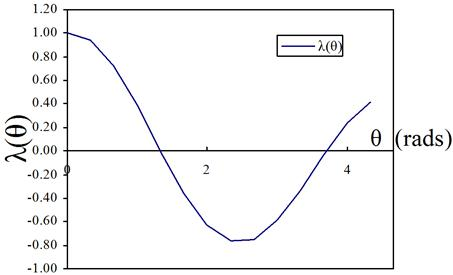Fig. 3. Calculated Lagrange multipliers for the two-dimensional model problem.

Both curves in Figure 4 indicate an algebraic convergence with Nλ. This behavior will be explained theoretically below.

Table 3. Converged values of the leading singular coefficients βj for Na=Nλ=13.

 j βj (approximate) βj (exact) 1 0.000000000000000 0.000000000000000 2 0.400000000000000 0.400000000000000 3 0.571428571428572 0.571428571428571 4 0.000000000000000 0.000000000000000 5 0.000000000000000 0.000000000000000 ... ............................... ...............................

The above behavior of the present technique was also demonstrated in previous implementations of the method, in both 2-D and 3-D problems [9-14].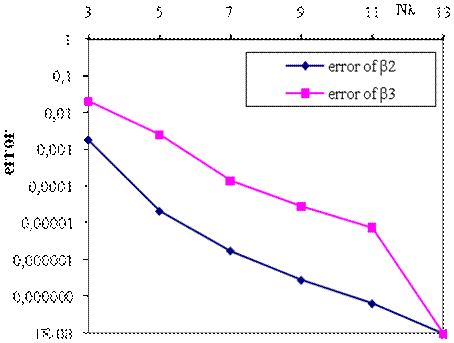Fig. 4. Approximation error for the singular coefficients β2 and β3, with Nλ; Na=13 and R=1 (two-dimensional model problem).

According to reference , if λ Hk(ΩAB), for some k≥1 and λh is the approximation of the Lagrange multiplier function with h being always the mesh-width, then there exist positive constants C and γ(0,1), independent of Nα and h such that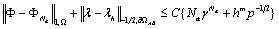(39)

where m=min{k, p+1} and Hk(ΩAB), kN, is the usual Sobolev space on boundary part AB, which contains functions that have k generalized derivatives in the space of the square integrable functions L2(Ω). Also, in  it was shown that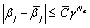(40)

Inequalities (39) and (40) clearly indicate exponential convergence of the method with respect to the number of singular functions Na and an algebraic convergence with the number of Lagrange multipliers Nλ. This fast convergence is visible in the results presented in Tables 1 and 2 and in the plot of Figure 4. In this study it is k=2 for the Sobolev space used. Also, quadratic basis functions were chosen (p=2). Now, if the two errors in (39) are forced to be equal to each other then we obtain(41)

Hence using (41) and the "optimal" pair Na=Nλ=13 we find that γ0.770.80 (0,1). Therefore, with this value of γ and by prescribing parameter Na, one can find Nλ from (41) and thus can have another pair of values. Using these values convergence of the method can be achieved.

4.2. Numerical Results for the 3-D Problem

In order to implement the SFBIM for the 3-D model problem, the boundary part SC is subdivided into standard 3-D boundary elements (having dimensions along z and θ directions only) as shown in Figure 5. Specifically, NE=Nz´Nθ elements are employed. Figure 5 shows the division of cylindrical boundary SC into elements. As it is indicated by equations (35) and (36), calculations of the integrals, finally take place in a 2-D space (Figure 5). On the same figure the bilinear basis functions are also presented. The total number of Lagrange multipliers is Nλ=(Nz+1)´(Nθ+1). The surface integrals are estimated using a 9-point Gaussian quadrature rule over each element.

For all computations presented we take Np=1 in (26), i.e. the EFIFs are approximated by polynomials of linear form. As explained earlier, this choice is made in order to force boundary integrals on SD and SE to vanish. In this way the integration in the vicinity of point singularities O and D is avoided. Now, with this choice of Np, the parameter N in (26) is determined to be equal to 1; choosing a value N > 1 will not yield any additional terms in (26). Systematic runs have been carried out for the model problem in order to study the effects of Na and Nλ and of the other parameters, on the numerical results.

The runs for different values of R included various combinations between Na and Nλ in an attempt to find the "ideal’’ combination of these parameters, for which the method converges. Calculations were made with different combinations of Nz and Nθ but the pair of Nz=2 and Nθ=2 indicated (in combination with a suitable value of Na) convergence of the method. Table 4 contains the values of the first two singular coefficients of A(a1)(z) (i.e. of a1,1 and a1,2) obtained with Nλ=9, R =0.01 and for different values of Na. Note that since Np+1=2, the step increment for Nap=(Np+1)Na in all the trials, is equal to 2. One may immediately observe very high rate of convergence with respect to Na and great accuracy in the values obtained, even up to the 14th decimal digit. Also, Table 5 shows the values of the first two EFIFs at the points z = 0.5 and 1, respectively, (i.e. of A(a1)(0.5) and A(a2)(1.0)) calculated for the same values of Nλ and R and for various values of Na.

The values of z were selected so that a comparison can be made between the SFBIM and the energy projection method presented in . We may observe, again, very fast convergence with respect to Na and high accuracy in the values obtained. In both Tables 4 and 5 convergence corresponds to the "optimal" combination of values Na=7, Nap=(Np+1)Na=14 and Nλ = 9. The value of the radius of the domain, for our 3-D model problem, is taken as R = 0.01. A model problem of the same geometry and the same dimensions was also adopted in , where a special finite element technique was implemented in order to solve it.Fig. 5. Division of cylindrical boundary SC into boundary elements.

Table 6 contains the converged approximate values of the singular coefficients obtained for R = 0.01 and for the "optimal" combination of Na and Nλ. One may observe that the converged values of the coefficients, obtained with the SFBIM, are practically the same with those contained in the exact form of the EFIFs which is known for this model problem as already explained above.

Table 4. Convergence of the leading singular coefficients αi,k with (Np+1)Na; Nλ = 9; Np =1; R = 0.01.a1,1 a1,2 10 0.99999999999993294 0.50000000000005129 12 1.00000000000000020 0.50000000000000766 14 1.00000000000000004 0.50000000000000001 16 0.99999999999998842 0.50000000000014344 18 0.99999999998523833 0.50000000001876197

Table 5. Convergence of the first two EFIFs A(a1)(z) and A(a2)(z) at z = 0.5 and at z=1.0, respectively, with (Np+1)Na; for Nλ = 9; R = 0.01.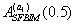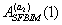10 1.25000000000006 1.50000000000008 12 1.25000000000000 1.50000000000001 14 1.25000000000000 1.50000000000000 16 1.25000000000106 1.50000000000113 18 1.25000000001046 1.50000000001140

Finally, Table 7 compares the values obtained by the SFBIM for R=0.01 and z=1.0 with the results given by the energy projection method , for the same values of R and z. The exact solution, for our 3-D model problem, is also presented on the same table. Clearly, the SFBIM gives results which are comparable with the exact values.

In fact, for the accuracy considered in this work, the approximate values obtained with the SFBIM, coincide with the exact values. Also, it is obvious that the SFBIM, is significantly more accurate than the finite element technique which is implemented in . This is because the SFBIM is a direct method and no post-processing is required. Also, the numerical error and the CPU time in the SFBIM, are much smaller than those observed in other numerical techniques, for this type of boundary value problems in Applied Mathematics.

Table 6. Converged values of the leading singular coefficients ai,k for R=0.01 and the "optimal" combination (Np+1)Na =14; Nλ =9; Np=1; N=1.

 i k ai,k 1 1 1.0000000000000004 1 2 0.5000000000000001 2 1 1.0000000000000003 2 2 0.5000000000000048 ... ... ................................... ... ... ...................................

Table 7. Comparison between the values obtained by the SFBIM and the energy projection method  for R = 0.01 and z = 1.0, for the first two EFIFs.

 i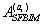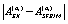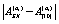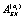1 1.500 0.000 1.499 0.001 1.500 2 1.500 0.000 1.500 0.000 1.500

In Figure 6 there is a graph presenting with "squares" the approximation of the first EFIF A(a1)(z)=A1(z) obtained at convergence, for which it is Nap =Na(Np+1)=14 and Nλ=9. On the same graph and with a solid line, the analytic form of the first EFIF A(a1)(z) is also presented. One may observe that, practically, the approximation of the polynomial A(a1)(z) coincides with the exact solution. Finally, the graph of Figure 7 shows how the error changes its value as Nap varies. In this case, the error is defined by using the infinity norm as follows: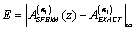.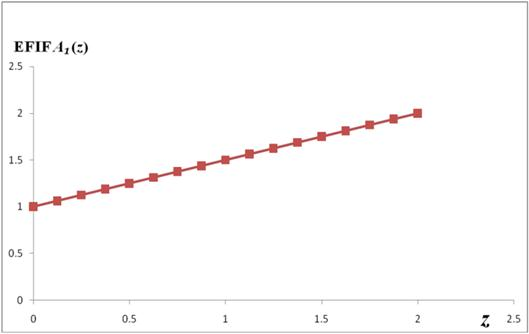Fig. 6. Graph of EFIF A(a1)(z); analytical (continuous line) and approximate ("squares").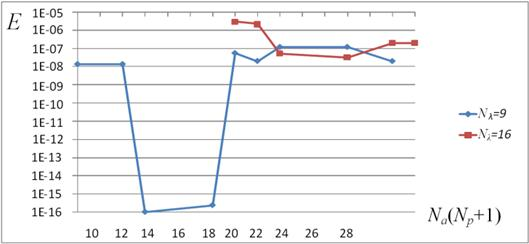Fig. 7. Graphical presentation of the error occurred in estimating EFIF A(a1)(z).

At Nap =Na(Np+1)=14, the error is minimized and it is of the order of 10-16. This value of error corresponds to the optimum combination of the parameters (i.e. for Na=7, Np=1, N=1 and Nλ=9). After a significant number of runs it was found that this is the minimum of the Ε vs. Nap graph for all values of Nλ. A general conclusion derived from these graphs is that the method requires a small number of boundary elements and that it exhibits very fast convergence.

5. Conclusions

The Singular Function Boundary Integral Method (SFBIM) has been formulated for both a 2-D elliptic boundary value problem with a point boundary singularity and a 3-D Laplace equation problem with a straight-edge boundary singularity. With this method the singular coefficients and the Edge Flux Inensity Functions (EFIFs), are the primary unknowns in the 2-D and the 3-D cases, respectively. The latter are approximated by polynomials, the coefficients of which are unknowns in the formulation.

Both the singular coefficients, of the two-dimensional local solution and the coefficients of the EFIFs, in the 3-D case, are calculated directly and not by post-processing of the solution. The implementation of the SFBIM to both a 2-D and a 3-D Laplacian model problems, yielded highly accurate results for the singular coefficients and the EFIFs and exhibited fast convergence, as in other two-dimensional applications [9-12] of the method. For the planar problem, the numerical results are favorably compared with the analytic solution. The numerical results for the 3-D case compare favorably with both the exact solution and those of the energy projection method .

Currently a particular version of the method is being investigated for other three-dimensional Laplace equation problems. This approach will aim at the better treatment of the inner sum in Eq. (26). Also, a hybrid method is being developed in which the advantages of both the SFBIM and the finite element techniques are being exploited for problems in Mechanics with boundary singularities.

Appendix

Herein we will show that 2(W jk)=0 and thus that the approximation of u also satisfies the Laplace equation. So, we consider, first, expression (28). Then, the explicit form of W jk is as follows: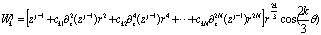So, we have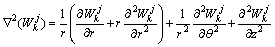whereandAlso,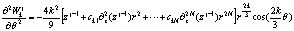andTherefore, after substituting in the expression of 2(W jk) the second order partial derivatives of W jk, with the above expansions and performing several algebraic operations, we obtain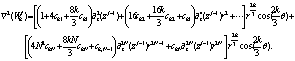It can be easily verified that all coefficients of the derivatives 2iz (zj-1), in the first brackets, cancel each other. Also, by considering the definition of parameter cki, the expression of 2(W jk) takes the formObviously, the algebraic operations inside the parentheses give us a zero result. Thus, the expression of 2 (W jk) shrinks to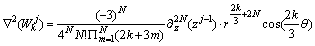which, of course, leads us to 2(W jk) = 0 if j-1 £ 2N+1 and since the maximum value of j is Np+1 we may say that W jk satisfy the 3-D Laplacian equation with the restriction Np £ 2N+1.

It is also easily shown that singular functions W jk satisfy the boundary conditions on SA and SB. Indeed, we have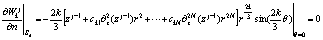and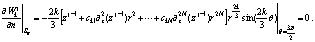References

1. Babuška I, Guo B (1986), "The h-p version of the finite element method –Part1: The basic approximation results", Comput. Mech. 1, 21-41.
2. Babuška I, Miller A (1984), "The post-processing approach in the finite element method – Part 1: Calculation of displacements, stresses and other higher order derivatives of the displacements", Int. J. Numer. Meth. Eng. 20, 1085-1109.
3. Babuška I, Miller A (1984), "The post-processing approach in the finite element method – Part 2: The calculation of the stress intensity factors", Int. J. Numer. Meth. Eng. 20, 1111-1129.
4. Beskos DE, Maier G (2003), Boundary Element Advances in Solid Mechanics, Springer, Wien, NY.
5. Brenner SC (1999), "Multigrid methods for the computation of singular solutions and stress intensity factors I: corner singularities", Math. Comput. 68, 559-583.
6. Christodoulou E, Elliotis M, Xenophontos C, Georgiou G (2012), "The Singular Function Boundary Integral Method for a 3-D Laplacian problem with a boundary straight edge singularity," Appl. Math. Comput. 219, 1073-1081.
7. Costabel M, Dauge M, Yosibash Z (2004), "A quasidual function method for extracting edge stress intensity functions", SIAM J. Math. Anal. 35 (5), 1177-1202.
8. Li ZC, Lu TT (2000), "Singularities and treatments of elliptic boundary value problems", Math. Comp. Model. 31, 97-145.
9. Elliotis M, Georgiou G, Xenophontos C (2002), "The solution of a Laplacian problem over an L-shaped domain with a singular function boundary integral method", Comm. Numer. Methods Eng. 18, 213-222.
10. Elliotis M, Georgiou G, Xenophontos C (2005), "Solving Laplacian problems with boundary singularities: A comparison of a singular function boundary integral method with the p/hp version of the finite element method", Appl. Math. Comput. 169, 485-499.
11. Elliotis M, Georgiou G, Xenophontos C (2005), "Solution of the planar Newtonian stick-slip problem with the singular function boundary integral method", Int. J. Numer. Meth. Fluids 48, 1000-1021.
12. Elliotis M, Georgiou G, Xenophontos C (2006), "The singular function boundary integral method for a two-dimensional fracture problem", Engineering Analysis with Boundary Elements 30, 100-106.
13. Elliotis M, Christodoulou E, Georgiou G, Xenophontos C (2010), "The Singular Function Boundary Integral Method for a 3-D Laplacian problem with an edge singularity," in Recent Developments in Boundary Element Methods (special edition to honor Prof. John Katsikadelis), E. J. Sapountzakis (Ed.), WIT Press, Southampton, 31-42.
14. Elliotis M, Charmpis D, Georgiou G (2014), "The Singular Function Boundary Integral Method for an elastic plane stress wedge beam problem with a point boundary singularity," Appl. Math. Comput. 248, 93-100.
15. Fritz J (1982), Partial Differential Equations, Springer -Verlang, Inc., NY.
16. Fung YC (1977), Foundations of solid mechanics, Prentice Hall, Inc., NJ.
17. Georgiou GC, Boudouvis A, Poullikkas A (1999), "Comparison of two methods for the computation of a singular solution in elliptic problems", J. Comp. Appl. Math., 79, 277-290.
18. Georgiou GC, Olson LG, Schultz WW, Sagan S (1989), "A singular finite element for Stoke’ s flow: the stick-slip problem", Int. J. Numer. Meth. Fluids 9, 1353-1367.
19. Georgiou GC, Olson LG, Smyrlis Y (1996), "A singular function boundary integral method for the Laplace equation", Comm. Numer. Methods Eng. 12, 127-134.
20. Grisvard P (1985), Elliptic problems in non-smooth domains, Pitman Publishers, UK.
21. Huber O, Nickel J, Kuhn G (1993), "On the decomposition of the J-integral for 3-D crack problems", Int. J. Fracture 64 (4), 339-348.
22. Katsikadelis JT, Armenakas AE (1984), "Plates on elastic Foundation by the Boundary Integral Equation Method", ASCE J. Eng. Mech. 110, 1086-1105.
23. Meda G, Messner TW, Sinclair GB, Solecki JS (1998), "Path-independent H integrals for three-dimensional fracture mechanics", Int. J. Fracture 94 (3), 217-234.
24. Olson LG, Georgiou GC, Schultz WW (1991), "An efficient finite element method for treating singularities in Laplace’s equation", J. Comp. Physics 96, 391-410.
25. Szabó B, Babuška I (1991), Finite Element Analysis, John Wiley & Sons, Inc., NY.
26. Trefftz E (1926), "Ein Gegenstuck zum Ritz’ schen Verfahren", Proc. Second Int. Cong. Appl. Mech., Zurch, 131-137.
27. Weiss D., Yosibash Z. (2014), "Uncertainty quantification for a 1D thermo-hyperelastic coupled problem using polynomial chaos projection and p-FEMs", Computers and Mathematics with Applications, 70, 1701-1720.
28. Xenophontos C, Elliotis M, Georgiou G (2006), "A singular function boundary integral method for Lapacian problems with boundary singularities", SIAM J. Sci. Comp. 28, 517-532.
29. Young DM, Gregory RT (1988), A survey of numerical mathematics, Dover Publications, Inc., NY.
30. Yosibash Z, Actis R, Szabo B (2002), "Extracting edge flux intensity functions for the Laplacian", Int. J. Numer. Meth. Eng. 53, 225-242.
31. Yosibash Z. (2011), Singularities in Elliptic Boundary Value Problems and Elasticity and their Connection with Failure Initiation, Springer, Berlin.
32. Yosibash Z., Shannon S. (2014), "Computing edge stress intensity functions (ESIFs) along circular 3-D edges", Engineering Fracture Mechanics, 117, 127-151.
33. Yosibash Z., Mittelman B. (2016), "A 3-D Failure Initiation Criterion from a Sharp V-notch Edge in Elastic Brittle Structures", European Journal of Mechanics - A/Solids, 60, 70-94.
34. Yosibash Z., Weiss D., Hartmann S. (2014), "High-order FEMs for thermo-hyperelasticity at finite strains", Computers and Mathematics with Applications, 67, 477-496.
35. Yosibash Z., Mittelman B. (2016), "A Revised Failure Criterion for Brittle Elastic Materials Under Mixed-mode Loading in 2-D", Theoretical and Applied Fracture Mechanics, Accepted.
36. Zaltzman T., Yosibash Z. (2011), "Vertex singularities associated with conical points for the 3-D Laplace equation", Numer. Methods PDEs 27, 662–679.

 Contents 1. 2. 2.1. 2.2. 3. 3.1. 3.2. 4. 4.1. 4.2. 5.
Article ToolsAbstractPDF(1464K)